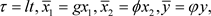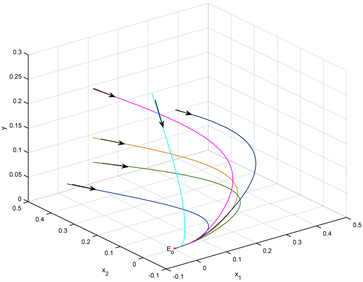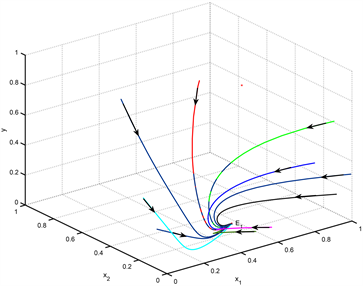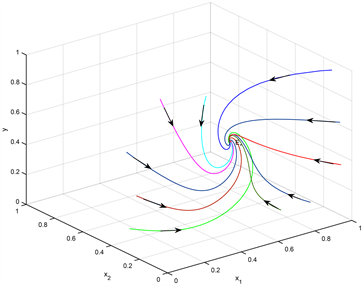﻿ 食饵具有扩散和恐惧效应的捕食–食饵模型的稳定性分析

# 食饵具有扩散和恐惧效应的捕食–食饵模型的稳定性分析Stability Analysis of Predator-Prey Model with Dispersal and Fear Effect

Abstract: In this paper, we propose a predator-prey model with fear effect and diffusion. By making full use of qualitative analysis, we obtain the detailed dynamic behavior of the system. We also study the influence of fear effect on the system and find that diffusion has a large effect on the persistence of predator and prey. Numerical simulation further demonstrates the feasibility of our theoretical conclusion.

1. 引言

$\begin{array}{l}\frac{\text{d}{x}_{1}}{\text{d}\tau }=\alpha {x}_{2}-\beta {x}_{1}+\epsilon \left({r}_{1}-{b}_{1}{x}_{1}-{a}_{1}y\right){x}_{1},\\ \frac{\text{d}{x}_{2}}{\text{d}\tau }=\beta {x}_{1}-\alpha {x}_{2}+\epsilon \left(-d{x}_{2}\right),\\ \frac{\text{d}y}{\text{d}\tau }=\epsilon \left({r}_{2}-\frac{{a}_{2}y}{{x}_{1}}\right)y,\end{array}$

$\begin{array}{l}\frac{\text{d}u}{\text{d}t}=\frac{{r}_{0}u}{1+kv}-du-a{u}^{2}-puv,\\ \frac{\text{d}v}{\text{d}t}=cpuv-mv,\end{array}$

2. 模型的建立

$\begin{array}{l}\frac{\text{d}{x}_{1}}{\text{d}t}=\alpha {x}_{2}-\beta {x}_{1}+{x}_{1}\left(\frac{{r}_{1}}{1+{k}_{1}y}-{d}_{1}-{b}_{1}{x}_{1}\right)-{a}_{1}{x}_{1}y,\\ \frac{\text{d}{x}_{2}}{\text{d}t}=\beta {x}_{1}-\alpha {x}_{2}-{d}_{2}{x}_{2},\\ \frac{\text{d}y}{\text{d}t}=\left({a}_{2}{x}_{1}-{d}_{3}-{b}_{2}y\right)y,\end{array}$ (1)$\begin{array}{l}\frac{\text{d}{x}_{1}}{\text{d}t}={x}_{2}-a{x}_{1}+{x}_{1}\left(\frac{\text{1}}{1+ky}-d-{x}_{1}\right)-{x}_{1}y,\\ \frac{\text{d}{x}_{2}}{\text{d}t}=b\left(c{x}_{1}-{x}_{2}\right),\\ \frac{\text{d}y}{\text{d}t}=f\left({x}_{1}-e-hy\right)y,\end{array}$ (2)3. 平衡点的局部稳定性

$\left\{\begin{array}{l}-a{x}_{1}+{x}_{2}+{x}_{1}\left(\frac{1}{1+ky}-{d}_{1}-{x}_{1}\right)-{x}_{1}y=0,\\ b\left(c{x}_{1}-{x}_{2}\right)=0,\\ f\left(x-e-hy\right)y=0,\end{array}$

$k\left(h+1\right){y}^{2}+\left(h+1+k\left(a+d+e-c\right)\right)y+a+d+e-c-1=0.$

$\begin{array}{c}\Delta ={\left(h+1+k\left(a+d+e-c\right)\right)}^{2}-4k\left(h+1\right)\left(a+d+e-c-1\right)\\ ={\left(h+1-k\left(a+d+e-c\right)\right)}^{2}+4k\left(h+1\right)>0.\end{array}$

(1) 系统(2)始终存在一个灭绝平衡点 ${E}_{0}\left(0,0,0\right)$

(a) 若 $c+1，那么 ${E}_{0}\left(0,0,0\right)$ 是局部渐近稳定的；

(b) 若 $c+1=a+d$${E}_{0}\left(0,0,0\right)$ 是鞍结点；

(c) 若 $c+1>a+d$${E}_{0}\left(0,0,0\right)$ 是不稳定的。

(2) 当 $c+1>a+d$，那么系统(2)有唯一个边界平衡点 ${E}_{1}\left({x}_{11},{x}_{22},0\right)$

(a) 若 $a+d，它是局部渐近稳定的；

(b) 若 $c+1=a+d+e$${E}_{1}\left({x}_{11},{x}_{22},0\right)$ 是鞍结点；

(c) 若 $c+1>a+d+e$${E}_{1}\left({x}_{11},{x}_{22},0\right)$ 是不稳定的。

(3) 当 $c+1>a+d+e$，那么系统(2)有唯一一个正平衡点 ${E}_{2}\left({x}_{1}^{*},c{x}_{1}^{*},{y}^{*}\right)$，且是局部渐近稳定的。

${J}_{E}=\left(\begin{array}{ccc}-a-d-2x-y+\frac{1}{1+ky}& 1& -x\left(1+k\right)\\ bc& -b& 0\\ fy& 0& f\left(-2hy-e+x\right)\end{array}\right).$

(1) 系统在平衡点 ${E}_{0}\left(0,0,0\right)$ 处的雅可比矩阵为

${J}_{{E}_{0}}=\left(\begin{array}{ccc}-a-d+1& 1& 0\\ bc& -b& 0\\ 0& 0& -fe\end{array}\right).$

${J}_{{E}_{0}}$ 的特征方程是

$\left(\lambda +fe\right)\left({\lambda }^{2}+\left(a+b+d-1\right)\lambda +\left(a+d-1-c\right)b\right)=0.$

$\begin{array}{l}{\lambda }_{2}=\frac{-\left(a+b+d-1\right)+\sqrt{{\left(a+b+d-1\right)}^{2}-4\left(a+d-1-c\right)}}{2},\\ {\lambda }_{3}=\frac{-\left(a+b+d-1\right)-\sqrt{{\left(a+b+d-1\right)}^{2}-4\left(a+d-1-c\right)}}{2}.\end{array}$

$c+1 时，有 ${\lambda }_{2}<0,{\lambda }_{3}<0$，因此 ${E}_{0}\left(0,0,0\right)$ 是局部渐近稳定的；当 $c+1>a+d$ 时，有 ${\lambda }_{2}>0,{\lambda }_{3}<0$，因此 ${E}_{0}\left(0,0,0\right)$ 是不稳定的；当 $c+1=a+d$，此时 ${\lambda }_{2}=0,{\lambda }_{3}<0$。为了确定 ${E}_{0}\left(0,0,0\right)$ 的类型，将系统(2)在 ${E}_{0}$ 处线性化，并将线性部分对角化。做变换

$\left(\begin{array}{c}{x}_{1}\\ {x}_{2}\\ y\end{array}\right)=\left(\begin{array}{ccc}\frac{1}{c}& 0& -\frac{1}{b}\\ 1& 0& 1\\ 0& 1& 0\end{array}\right)\left(\begin{array}{c}{X}_{1}\\ {X}_{2}\\ Y\end{array}\right),$

$\left\{\begin{array}{l}{\stackrel{˙}{X}}_{1}=-\frac{b{X}_{1}^{2}}{\left(b+c\right)c}-\frac{c{Y}^{2}}{\left(b+c\right)c}+\frac{2{X}_{1}Y}{b+c}-\frac{b\left(1+k\right){X}_{1}{X}_{2}}{b+c}+\frac{c\left(1+k\right){X}_{2}Y}{b+c}+O\left({|{X}_{1},{X}_{2},Y|}^{3}\right),\\ {\stackrel{˙}{X}}_{2}=-fe{X}_{2}-fh{X}_{2}^{2}-\frac{f}{b}{X}_{2}Y+\frac{f}{c}{X}_{1}{X}_{2}+O\left({|{X}_{1},{X}_{2},Y|}^{3}\right),\\ \stackrel{˙}{Y}=-\left(b+c\right)Y+\frac{b{X}_{1}^{2}}{\left(b+c\right)c}+\frac{c{Y}^{2}}{\left(b+c\right)c}-\frac{2{X}_{1}Y}{b+c}-\frac{c\left(1+k\right){X}_{2}Y}{b+c}+\frac{b\left(1+k\right){X}_{1}{X}_{2}}{b+c}+O\left({|{X}_{1},{X}_{2},Y|}^{3}\right),\end{array}$

(2) 系统(2)在平衡点 ${E}_{1}\left({x}_{11},{x}_{22},0\right)$ 处的雅可比矩阵为

${J}_{{E}_{0}}=\left(\begin{array}{ccc}-{x}_{11}-c& 1& -{x}_{11}\left(1+k\right)\\ bc& -b& 0\\ 0& 0& f\left({x}_{11}-e\right)\end{array}\right).$

$\begin{array}{l}{\lambda }_{1}=\frac{-\left(b+c+{x}_{11}\right)+\sqrt{{\left(b+c+{x}_{11}\right)}^{2}-4b{x}_{11}}}{2}<0,\\ {\lambda }_{2}=\frac{-\left(b+c+{x}_{11}\right)-\sqrt{{\left(b+c+{x}_{11}\right)}^{2}-4b{x}_{11}}}{2}<0,\\ {\lambda }_{3}=f\left({x}_{11}-e\right),\end{array}$

$c+1-e，有 ${\lambda }_{3}<0$，故 ${E}_{1}\left({x}_{11},{x}_{22},0\right)$ 是局部渐近稳定的；当 $c+1-e>a+d$ 时， ${\lambda }_{3}>0$，因此 ${E}_{1}\left({x}_{11},{x}_{22},0\right)$ 是不稳定的；当 $c+1-e=a+d$ 时，有 ${\lambda }_{3}=0$。类似于(1)中的方法，先做变换 $\left({x}_{1},{x}_{2},y\right)=\left({X}_{1},{X}_{2},Y\right)+\left(e,ce,0\right)$，将 ${E}_{1}$ 平移到原点并作泰勒展开得到如下系统

$\left\{\begin{array}{l}{\stackrel{˙}{X}}_{1}=-\left(c+e\right){X}_{1}\text{+}{X}_{2}-e\left(k+1\right)Y-{X}_{1}^{2}-\left(k+1\right){X}_{1}Y+e{k}^{2}{Y}^{2}+O\left({|{X}_{1},{X}_{2},Y|}^{3}\right),\\ {\stackrel{˙}{X}}_{2}=bc{X}_{1}-b{X}_{2}+O\left({|{X}_{1},{X}_{2},Y|}^{3}\right),\\ \stackrel{˙}{Y}=f{X}_{1}Y-fh{Y}^{2}+O\left({|{X}_{1},{X}_{2},Y|}^{3}\right),\end{array}$ (3)

$\left(\begin{array}{c}{X}_{1}\\ {X}_{2}\\ Y\end{array}\right)=\left(\begin{array}{ccc}1& 1& -\left(k+1\right)\\ {\lambda }_{1}+c+e& {\lambda }_{2}+c+e& -c\left(k+1\right)\\ 0& 0& 1\end{array}\right)\left(\begin{array}{c}{U}_{1}\\ {U}_{2}\\ V\end{array}\right),$

$\left\{\begin{array}{l}{\stackrel{˙}{U}}_{1}={\lambda }_{1}{U}_{1}+O\left({|{U}_{1},{U}_{2},V|}^{2}\right),\\ {\stackrel{˙}{U}}_{2}={\lambda }_{2}{U}_{2}+O\left({|{U}_{1},{U}_{2},V|}^{2}\right),\\ \stackrel{˙}{V}=-f\left(h+k+1\right){V}^{2}+f{U}_{1}V+f{U}_{2}V+O\left({|{U}_{1},{U}_{2},V|}^{3}\right),\end{array}$

(3) 系统(2)在 ${E}_{2}\left({x}_{1}^{*},c{x}_{1}^{*},y\right)$ 处的雅可比矩阵是

${J}_{{E}_{2}}=\left(\begin{array}{ccc}-c-{x}_{1}^{*}& 1& -{x}_{1}^{*}\left(1+\frac{k}{{\left(1+k{y}^{*}\right)}^{2}}\right)\\ bc& -b& 0\\ f{y}^{*}& 0& -fh{y}^{*}\end{array}\right),$

${\lambda }^{3}+{a}_{1}{\lambda }^{2}+{a}_{2}\lambda +{a}_{3}=0,$

$\begin{array}{l}{a}_{1}=fh{y}^{*}+b+c+{x}_{1}^{*}>0,\\ {a}_{2}=fh{y}^{*}\left(b+c+{x}_{1}^{*}\right)+b{x}_{1}^{*}+f{x}_{1}^{*}y\left(1+\frac{k}{{\left(1+k{y}^{*}\right)}^{2}}\right)>0,\\ {a}_{3}=bfh{x}_{1}^{*}{y}^{*}+bf{x}_{1}^{*}{y}^{*}\left(1+\frac{k}{{\left(1+k{y}^{*}\right)}^{2}}\right)>0,\end{array}$

$\begin{array}{c}{a}_{1}{a}_{2}-{a}_{3}=\left(fh{y}^{*}+b+c+{x}_{1}^{*}\right)\left(fh{y}^{*}\left(b+c+{x}_{1}^{*}\right)+b{x}_{1}^{*}+f{x}_{1}^{*}y\left(1+\frac{k}{{\left(1+k{y}^{*}\right)}^{2}}\right)\right)\\ \text{\hspace{0.17em}}-bfh{x}_{1}^{*}{y}^{*}-bf{x}_{1}^{*}{y}^{*}\left(1+\frac{k}{{\left(1+k{y}^{*}\right)}^{2}}\right)\\ =\left(b+c+{x}_{1}^{*}\right)b{x}_{1}^{*}+fh{y}^{*}\left(b+c+{x}_{1}^{*}\right)\left(b+c+{x}_{1}^{*}+fh{y}^{*}\right)\\ \text{\hspace{0.17em}}+f{x}_{1}^{*}y\left(1+\frac{k}{{\left(1+k{y}^{*}\right)}^{2}}\right)\left(fh{y}^{*}+c+{x}_{1}^{*}\right)\\ >0.\end{array}$Table 1. The existence and stability of equilibrium of system (2)

4. 全局稳定性

$V={x}_{1}+\frac{1}{b}{x}_{2}+\frac{1}{f+1}y,$

$\begin{array}{c}{D}^{+}V\left(t\right)=-{x}_{1}^{2}-\frac{f}{f+1}\left(e+hy\right)y+\left(\frac{1}{1+ky}-a-d+c-\frac{1}{f+1}y\right){x}_{1}\\ \le -{x}_{1}^{2}-\frac{f}{f+1}\left(e+hy\right)y+\left(1+c-a-d-\frac{1}{f+1}y\right){x}_{1}\\ \le 0.\end{array}$

$1+c-a-d<0$ 时，对任意的 ${x}_{1},{x}_{2},y$，都有 ${D}^{+}V\left(t\right)<0$，此外，当且仅当在边界平衡点 ${E}_{0}\left(0,0,0\right)$ 处有 ${D}^{+}V\left(t\right)=0$，这满足李雅普诺夫渐近稳定性定理 ，故 ${E}_{0}$ 是全局渐近稳定的。

$V=\frac{1}{{x}_{22}}\left({x}_{1}-{x}_{1}^{*}-{x}_{1}^{*}\mathrm{ln}\frac{{x}_{1}}{{x}_{1}^{*}}\right)+\frac{1}{bc{x}_{11}}\left({x}_{2}-{x}_{2}^{*}-{x}_{2}^{*}\mathrm{ln}\frac{{x}_{2}}{{x}_{2}^{*}}\right)+\frac{1}{f{x}_{22}}y,$

$\begin{array}{c}\stackrel{˙}{V}=\frac{1}{{x}_{22}}\cdot \frac{{x}_{1}-{x}_{11}}{{x}_{1}}\cdot {\stackrel{˙}{x}}_{1}+\frac{1}{bc{x}_{11}}\cdot \frac{{x}_{2}-{x}_{22}}{{x}_{2}}\cdot {\stackrel{˙}{x}}_{2}+\frac{1}{f{x}_{22}}\cdot \stackrel{˙}{y}\\ =\frac{1}{{x}_{22}}\left({x}_{1}-{x}_{11}\right)\left(\frac{{x}_{2}}{{x}_{1}}-\frac{{x}_{22}}{{x}_{11}}-\left({x}_{1}-{x}_{11}\right)+\frac{1}{1+ky}-1-y\right)+\frac{1}{{x}_{11}}\left({x}_{2}-{x}_{22}\right)\left(\frac{{x}_{1}}{{x}_{2}}-\frac{{x}_{11}}{{x}_{22}}\right)+\frac{1}{{x}_{22}}\left({x}_{1}-e-hy\right)y\\ =\left(2-\frac{{x}_{11}{x}_{2}}{{x}_{1}{x}_{22}}-\frac{{x}_{1}{x}_{22}}{{x}_{11}{x}_{2}}\right)+\frac{1}{{x}_{22}}\left({x}_{11}-e\right)y-\frac{1}{{x}_{22}}{\left({x}_{1}-{x}_{11}\right)}^{2}-\frac{h}{{x}_{22}}{y}^{2}+\frac{k}{{x}_{22}\left(1+ky\right)}\left({x}_{1}-{x}_{11}\right)\left(-y\right)\\ \le \frac{k}{2{x}_{22}\left(1+ky\right)}\left\{{\left({x}_{1}-{x}_{11}\right)}^{2}+{y}^{2}\right\}-\frac{1}{{x}_{22}}{\left({x}_{1}-{x}_{11}\right)}^{2}-\frac{h}{{x}_{22}}{y}^{2}+\left(2-\frac{{x}_{11}{x}_{2}}{{x}_{1}{x}_{22}}-\frac{{x}_{1}{x}_{22}}{{x}_{11}{x}_{2}}\right)+\frac{1}{{x}_{22}}\left({x}_{11}-e\right)y\\ \le \frac{k}{2{x}_{22}}\left\{{\left({x}_{1}-{x}_{11}\right)}^{2}+{y}^{2}\right\}-\frac{1}{{x}_{22}}{\left({x}_{1}-{x}_{11}\right)}^{2}-\frac{h}{{x}_{22}}{y}^{2}+\left(2-\frac{{x}_{11}{x}_{2}}{{x}_{1}{x}_{22}}-\frac{{x}_{1}{x}_{22}}{{x}_{11}{x}_{2}}\right)+\frac{1}{{x}_{22}}\left({x}_{11}-e\right)y\\ =\frac{1}{{x}_{22}}\left\{\left(\frac{k}{2}-1\right){\left({x}_{1}-{x}_{11}\right)}^{2}+\left(\frac{k}{2}-h\right){y}^{2}\right\}+\left(2-\frac{{x}_{11}{x}_{2}}{{x}_{1}{x}_{22}}-\frac{{x}_{1}{x}_{22}}{{x}_{11}{x}_{2}}\right)+\frac{1}{{x}_{22}}\left({x}_{11}-e\right)y.\end{array}$

$c+1-e，即 ${x}_{11}，又当 $a>0$ 时， $2-a-\frac{1}{a}\le 0$ 恒成立。故对任意的 ${x}_{1},{x}_{2},y$ 都有 $\stackrel{˙}{V}\le \text{0}$，当且仅当在 ${E}_{1}\left({x}_{11}^{*},c{x}_{11}^{*},0\right)$ 处等号取到。利用李雅普诺夫渐近稳定性定理 ，故 ${E}_{1}$ 是全局渐近稳定的。

$V={k}_{1}\left({x}_{1}-{x}_{1}^{*}-{x}_{1}^{*}\mathrm{ln}\frac{{x}_{1}}{{x}_{1}^{*}}\right)+{k}_{2}\left({x}_{2}-{x}_{2}^{*}-{x}_{2}^{*}\mathrm{ln}\frac{{x}_{2}}{{x}_{2}^{*}}\right)+{k}_{3}\left(y-{y}^{*}-{y}^{*}\mathrm{ln}\frac{y}{{y}^{*}}\right)$

$\begin{array}{c}\frac{\text{d}V}{\text{d}t}=\frac{\partial V}{\partial {x}_{1}}\cdot \frac{\text{d}{x}_{1}}{\text{d}t}+\frac{\partial V}{\partial {x}_{2}}\cdot \frac{\text{d}{x}_{2}}{\text{d}t}+\frac{\partial V}{\partial y}\cdot \frac{\text{d}y}{\text{d}t}\\ ={k}_{1}\left({x}_{1}-{x}_{1}^{*}\right)\left(\frac{{x}_{2}}{{x}_{1}}-a+\frac{1}{1+ky}-d-{x}_{1}-y\right)+{k}_{2}\left({x}_{2}-{x}_{2}^{*}\right)\cdot \left(bc\cdot \frac{{x}_{1}}{{x}_{2}}-b\right)\\ \text{\hspace{0.17em}}+{k}_{3}\left(y-{y}^{*}\right)\cdot f\cdot \left({x}_{1}-e-hy\right)\\ ={k}_{1}\left({x}_{1}-{x}_{1}^{*}\right)\left(\frac{{x}_{2}}{{x}_{1}}-\frac{{x}_{2}^{*}}{{x}_{1}^{*}}\right)+{k}_{2}\left({x}_{2}-{x}_{2}^{*}\right)\cdot b\cdot c\cdot \left(\frac{{x}_{1}}{{x}_{2}}-\frac{{x}_{1}^{*}}{{x}_{2}^{*}}\right)\\ \text{\hspace{0.17em}}+{k}_{1}\left({x}_{1}-{x}_{1}^{*}\right)\left(\frac{1}{1+ky}-\frac{1}{1+k{y}^{*}}+{x}_{1}^{*}-{x}_{1}+{y}^{*}-y\right)+{k}_{3}f\left(y-{y}^{*}\right)\cdot \left({x}_{1}-{x}_{1}^{*}-h\left(y-{y}^{*}\right)\right)\end{array}$

$\begin{array}{c}\frac{\text{d}V}{\text{d}t}=\left({x}_{1}-{x}_{1}^{*}\right)\left(\frac{{x}_{2}}{{x}_{2}^{*}{x}_{1}}-\frac{1}{{x}_{1}^{*}}\right)+\left({x}_{2}-{x}_{2}^{*}\right)\left(\frac{{x}_{1}}{{x}_{1}^{*}{x}_{2}}-\frac{1}{{x}_{2}^{*}}\right)-\frac{{\left({x}_{1}-{x}_{1}^{*}\right)}^{2}}{{x}_{2}^{*}}+\frac{k}{{x}_{2}^{*}}\frac{\left({x}_{1}-{x}_{1}^{*}\right)\left({y}^{*}-y\right)}{\left(1+ky\right)\left(1+k{y}^{*}\right)}-\frac{h}{{x}_{2}^{*}}{\left(y-{y}^{*}\right)}^{2}\\ =\left(2-\frac{{x}_{1}^{*}{x}_{2}}{{x}_{2}^{*}{x}_{1}}-\frac{{x}_{2}^{*}{x}_{1}}{{x}_{1}^{*}{x}_{2}}\right)+\frac{1}{{x}_{2}^{*}}\left(-{\left({x}_{1}-{x}_{1}^{*}\right)}^{2}+\frac{k\left({x}_{1}-{x}_{1}^{*}\right)\left({y}^{*}-y\right)}{\left(1+ky\right)\left(1+k{y}^{*}\right)}-h{\left(y-{y}^{*}\right)}^{2}\right)\\ \le \frac{1}{{x}_{2}^{*}}\left\{\left(\frac{k}{2}-1\right){\left({x}_{1}-{x}_{1}^{*}\right)}^{2}+\left(\frac{k}{2}-h\right){\left(y-{y}^{*}\right)}^{2}\right\},\end{array}$

5. 扩散和恐惧效应对系统(1)的影响

5.1. 恐惧效应对食饵与捕食者密度的影响

$\begin{array}{l}\frac{\text{d}\stackrel{˜}{y}}{\text{d}{k}_{1}}=\frac{{b}_{1}}{{r}_{1}{a}_{1}}\cdot \frac{\text{d}{y}^{*}}{\text{d}{k}_{1}}=\frac{\text{d}{y}^{*}}{\text{d}k}=\frac{\sqrt{\Delta }+\left(a+d+e-c-2\right)k-h-1}{2\sqrt{\Delta }{k}^{2}},\\ \frac{\text{d}{\stackrel{˜}{T}}_{1}}{\text{d}{k}_{1}}=\frac{{r}_{1}}{{b}_{1}}\cdot \frac{\text{d}{T}_{1}}{\text{d}{k}_{1}}=\frac{{r}_{1}^{2}{a}_{1}}{{b}_{1}^{2}}\cdot \frac{\text{d}{T}_{1}}{\text{d}k}=\frac{{r}_{1}^{2}{a}_{1}}{{b}_{1}^{2}}\cdot \frac{\text{d}\left({x}_{1}^{*}+c{x}_{1}^{*}\right)}{\text{d}k}\\ \text{\hspace{0.17em}}\text{\hspace{0.17em}}\text{\hspace{0.17em}}\text{\hspace{0.17em}}\text{\hspace{0.17em}}\text{\hspace{0.17em}}=\frac{{r}_{1}^{2}{a}_{1}}{{b}_{1}^{2}}\cdot \frac{h\left(c+1\right)\left(\sqrt{\Delta }+\left(a+d+e-c-2\right)k-h-1\right)}{2\sqrt{\Delta }{k}^{2}},\end{array}$

5.2. 扩散对食饵与捕食者密度的影响

$\begin{array}{l}\frac{\text{d}\stackrel{˜}{y}}{\text{d}\beta }=\frac{{b}_{1}}{{a}_{1}{r}_{1}}\cdot \frac{\text{d}{y}^{*}}{\text{d}\beta }=\frac{{b}_{1}}{{a}_{1}{r}_{1}}\cdot \frac{\text{d}{y}^{*}}{\text{d}a}\cdot \frac{\text{d}a}{\text{d}\beta }=\frac{{b}_{1}}{{a}_{1}{r}_{1}^{2}}\cdot \frac{\left(a+d+e-c\right)k-h-1-\sqrt{\Delta }}{2\sqrt{\Delta }\left(h+1\right)},\\ \frac{\text{d}{\stackrel{˜}{T}}_{1}}{\text{d}\beta }=\frac{{r}_{1}}{{b}_{1}}\cdot \frac{\text{d}{T}_{1}}{\text{d}\beta }=\frac{{r}_{1}}{{b}_{1}}\cdot \frac{\text{d}{T}_{1}}{\text{d}a}\cdot \frac{\text{d}a}{\text{d}\beta }=\frac{1}{{b}_{1}}\cdot \frac{h\left(c+1\right)\left(\left(a+d+e-c\right)k-h-1-\sqrt{\Delta }\right)}{2\sqrt{\Delta }\left(h+1\right)},\\ \frac{\text{d}\stackrel{˜}{y}}{\text{d}\alpha }=\frac{{b}_{1}}{{a}_{1}{r}_{1}}\cdot \frac{\text{d}{y}^{*}}{\text{d}\alpha }=\frac{{b}_{1}}{{a}_{1}{r}_{1}}\cdot \frac{1}{2\left(h+1\right)}\frac{\beta {d}_{2}}{{r}_{1}{\left(\alpha +{d}_{2}\right)}^{2}}\cdot \frac{\sqrt{\Delta }+h+1-\left(a+d+e-c\right)k}{\sqrt{\Delta }},\\ \frac{\text{d}{\stackrel{˜}{T}}_{1}}{\text{d}\alpha }=\frac{{r}_{1}}{{b}_{1}}\cdot \frac{\text{d}{T}_{1}}{\text{d}\alpha }=\frac{{r}_{1}}{{b}_{1}}\cdot \left(1+c\right)\cdot h\cdot \frac{\text{d}{y}^{*}}{\text{d}\alpha }=\frac{\left(1+c\right)h}{2{a}_{1}{r}_{1}\left(h+1\right)}\frac{\beta {d}_{2}}{{\left(\alpha +{d}_{2}\right)}^{2}}\cdot \frac{\sqrt{\Delta }+h+1-\left(a+d+e-c\right)k}{\sqrt{\Delta }},\end{array}$

${E}_{2}\left({x}_{1}^{*},c{x}_{1}^{*},{y}^{*}\right)$ 存在时，可推出条件 $h+1+k\left(a+d+e-c\right)<\sqrt{\Delta } 成立，通过放缩法可以得到 $\frac{\text{d}\stackrel{˜}{y}}{\text{d}\beta }<-\frac{{b}_{1}}{{a}_{1}{r}_{1}^{2}}\cdot \frac{1}{\sqrt{\Delta }}<0,\frac{\text{d}{\stackrel{˜}{T}}_{1}}{\text{d}\beta }<0,\frac{\text{d}\stackrel{˜}{y}}{\text{d}\alpha }>\frac{{b}_{1}}{{a}_{1}{r}_{1}}\cdot \frac{\beta {d}_{2}}{{r}_{1}{\left(\alpha +{d}_{2}\right)}^{2}}\cdot \frac{1}{\sqrt{\Delta }}>0,\frac{\text{d}{\stackrel{˜}{T}}_{1}}{\text{d}\alpha }>0$

6. 数值模拟Figure 1. Global asymptotic stability and the time series of E0Figure 2. Global asymptotic stability and the time series of ${E}_{1}$ with $k\le \mathrm{max}\left\{2,2h\right\}$Figure 3. Global asymptotic stability and the time series of ${E}_{2}$ with $k\le \mathrm{max}\left\{2,2h\right\}$

7. 结论

 Skellem, J.D. (1951) Dispersal in Theoretical Population. Biometrika, 38, 196-216.
https://doi.org/10.1093/biomet/38.1-2.196

 Levin, S.A. (1974) Dispersal and Population Interactions. The American Naturalist, 108, 207-228.
https://doi.org/10.1086/282900

 Freedman, H.I. and Waltman, D. (1977) Mathematical Models of Population Interactions with Dispersal: Stability of Two Habitats with and without a Predator. SIAM Journal on Applied Mathematics, 32, 631-648.
https://doi.org/10.1137/0132052

 Holt, R.D. (1985) Population Dynamics in Two-Patch Environments: Some Anomalous Consequences of an Optimal Habitat Distribution. Theoretical Population Biology, 28, 181-208.
https://doi.org/10.1016/0040-5809(85)90027-9

 Lu, Z. and Takeuchi, Y. (1993) Global Asymptotic Behavior in Single-Species Discrete Diffusion Systems. Journal of Mathematical Biology, 32, 67-77.
https://doi.org/10.1007/BF00160375

 Chen, F.D., Chen, L.J. and Xie, X.D. (2009) On a Leslie-Gower Predator-Prey Model Incorporating a Prey Refuge. Nonlinear Analysis: Real World Applications, 10, 2904-2908.
https://doi.org/10.1016/j.nonrwa.2008.09.009

 Wu, H., Wang, Y.S., Li, Y. and De Angelis, D.L. (2020) Dispersal Asymmetry in a Two-Patch System with Source-Sink Populations. Theoretical Population Biology, 131, 54-65.
https://doi.org/10.1016/j.tpb.2019.11.004

 Huang, R., Wang, Y.S. and Wu, H. (2020) Population Abundance in Predator-Prey Systems with Predators Dispersal between Two Patches. Theoretical Population Biology, 135, 1-8.
https://doi.org/10.1016/j.tpb.2020.06.002

 Liu, Z.G. (2018) The Effect of Prey Refuge in a Patchy Leslie-Gower Predation System. Acta Ecological Sinica, 38, 2958-2964.
https://doi.org/10.5846/stxb201704130654

 Wang, X.Y., Zanette, L.N. and Zou, X.F. (2016) Modelling the Fear Effect in Predator-Prey Interactions. Journal of Mathematical Biology, 73, 1179-1204.
https://doi.org/10.1007/s00285-016-0989-1

 Pal, S., .Pal, N. and Samanta, S. (2019) Effect of Hunting Cooperation and Fear in a Predator-Prey Model. Ecological Complexity, 39, 1-18.
https://doi.org/10.1016/j.ecocom.2019.100770

 Zhang, H.S., Cai, Y.L., Fu, S.M. and Wang, W.M. (2019) Impact of the Fear Effect in a Prey-Predator Model Incorporating a Prey Refuge. Applied Mathematics and Computation, 356, 328-337.
https://doi.org/10.1016/j.amc.2019.03.034

 Das, M. and Samanta, G.P. (2021) A Prey-Predator Fractional Order Model with Fear Effect and Group Defense. International Journal of Dynamics and Control, 9, 334-349.
https://doi.org/10.1007/s40435-020-00626-x

 Pal, S., Majhi, S., Mandal, S. and Pal, N. (2019) Role of Fear in a Predator-Prey Model with Beddington-DeAngelis Functional Response. Zeitschrift für Naturforschung, 74, 581-595.
https://doi.org/10.1515/zna-2018-0449

 Kundu, K., Pal, S. and Samanta, S. (2019) Impact of Fear Effect in a Discrete-Time Predator-Prey System. Bulletion Calcutta Mathmatical Society, 110, 245-264.

 Pandy, P., Pal, N., Samanta, S. and Chattopadhyay, J. (2019) A Three Species Food Chain Model with Fear Induced Trophic Cascade. International Journal of Applied and Computational Mathematics, 5, Article No. 100.
https://doi.org/10.1007/s40819-019-0688-x

 Wang, X.Y. and Zou, X.F. (2017) Modeling the Fear Effect in Predator-Prey Interactions with Adaptive Avoidance of Predators. Bulletin of Mathematical Biology, 79, 1325-1359.
https://doi.org/10.1007/s11538-017-0287-0

 张芷芬, 丁同仁, 黄文灶, 董镇喜. 微分方程定性理论[M]. 北京: 科学出版社, 2014.

 廖晓昕. 稳定性的理论、方法和应用[M]. 第2版. 武汉: 华中科技大学出版社, 2010.

 陈兰荪. 数学生态学模型与研究方法[M]. 第2版. 北京: 科学出版社, 2017.

Top